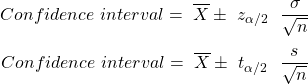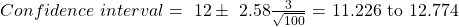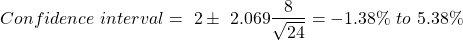Concept 12: Calculating Confidence Intervals | IFT World
101 Concepts for the Level I Exam

# Concept 12: Calculating Confidence IntervalsTo calculate a confidence interval for a population mean, follow these steps:

Refer to the table below and select t statistic or z statistic as per the scenario.

 Sampling from Small sample size Large sample size Normal distribution Variance known z z Variance unknown t t (or z) Non–normal distribution Variance known NA z Variance unknown NA t (or z)

Use the following formulae to calculate the confidence interval:For a Z distribution,

90% confidence à critical value = 1.65

95% confidence à critical value = 1.96

99% confidence à critical value = 2.58

You take a random sample of 100 large cap stocks. The average returns of these stocks for the past year is 12%. Assume that the average returns for all large-cap stocks in the economy follow a normal distribution with a standard deviation of 3%. Construct a 99% confidence interval for the average return all large-cap stocks for the past year.

Solution:

Since the population variance is known (the standard deviation of all large cap stocks), we will use Z statistic.

For confidence level of 99%, 1% error in both tails i.e. 0.5% (0.005) in one tail. Zα/2 = Z0.005 = 2.58 (From Z-table)

The confidence interval can be calculated asYou construct a sample of monthly returns of Stock A for the past two years. The stock has a mean return of 2% and a standard deviation of 8%. Compute the 95% confidence interval for the average monthly returns for this stock.

Solution:

Since the population variance is unknown (the variance of monthly returns of Stock A over its entire history, we only have data for the past two years) we will use t statistic.

Degrees of freedom = 24  – 1 = 23 (two years = 24 months)

For confidence level of 95%, 5% error in both tails, i.e. 2.5%(0.025) in one tail  tα/2 = t24, 0.025  = 2.069

The confidence interval can be calculated as:Crash Courses for November CFA Level I, II exams are coming soon!
This is default text for notification bar